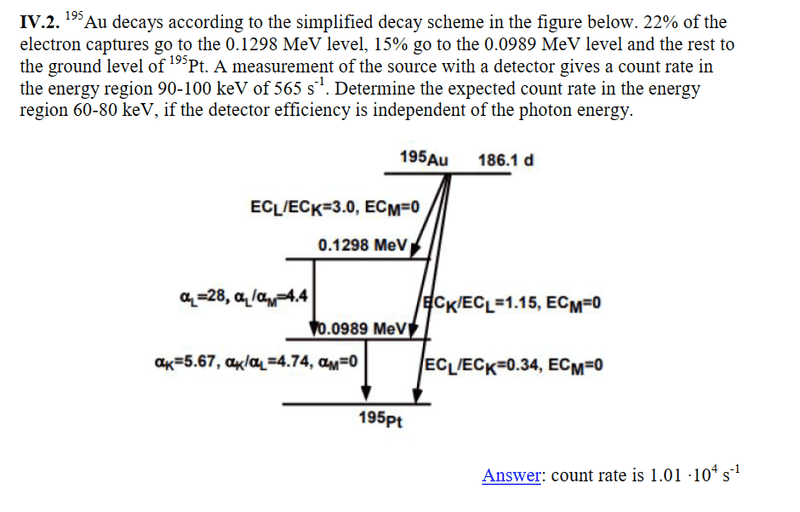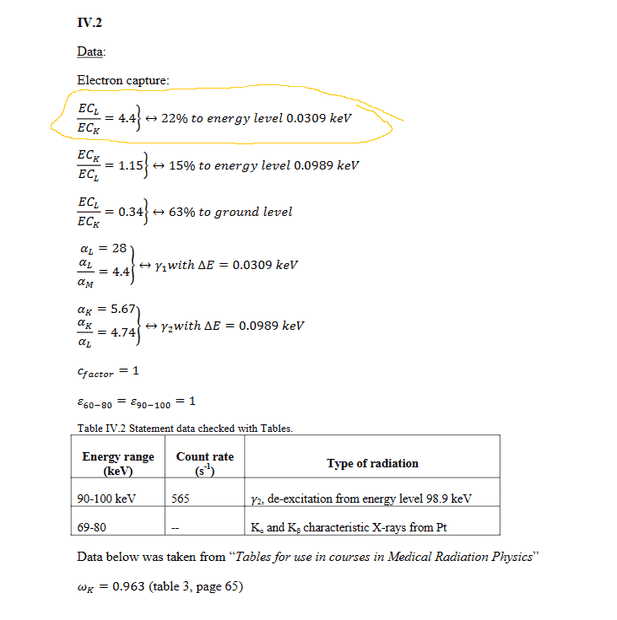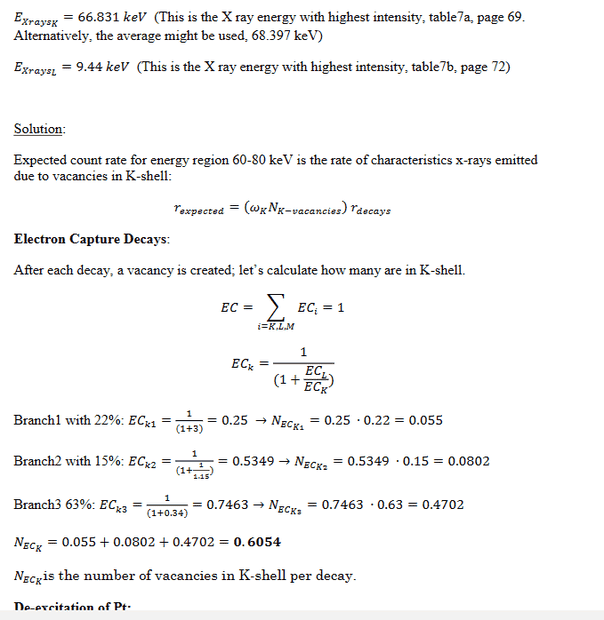# Radiation Physics - Decay Diagram

Graham87
Homework Statement:
See picture
Relevant Equations:
See pictureIn the solution below it says 22% goes to 0.0309keV. From the diagram above I interpret 22% goes to 0.1298keV with EC(L)/EC(K)=3.0 and not 4.4. Why is that wrong ?

Thanks alot!Last edited:

Homework Helper
Gold Member
2022 Award
View attachment 322938
In the solution below it says 22% goes to 0.0309keV. From the diagram above I interpret 22% goes to 0.1298keV with EC(L)/EC(K)=3.0 and not 4.4. Why is that wrong ?
View attachment 322939
Not sure how much I can help but there are some mistakes worth (IMO) mentioning.

Two excited nuclear energy levels (of Pt-125) are given as 0.1298MeV (=129.8keV) and 0.0989MeV (=98.9keV). Note that the difference between these is 0.0309MeV (= 30.9keV).

But there are then various references (including in the model-answer) to ‘0.1298keV’, ‘0.0989keV’ and ‘0.0309keV’. It appears that units are very messed up.

Also, the model-answer refers to an ‘energy level of 0.0309keV’. Even after correcting the unit, this value is not an energy level; it is the transition energy between the two excited nuclear states. What the model answer is telling you is that for each electron-capture, there are two possible nuclear emissions: a ##\gamma## photon of energy 30.9keV or one of 98.9keV. Note that only one of these is in the energy-range of interest.

I’m not familiar with some of the symbols used (despite several years of being a medical physicist in radiotherapy a long time ago). But I would suggest that you check the energies of characteristic X-rays for Pt-125 to see which ones are in the energy range of interest.

Edit: several minor changes.

Last edited:
•Graham87
Graham87
Not sure how much I can help but there are some mistakes worth (IMO) mentioning.

Two excited nuclear energy levels (of Pt-125) are given as 0.1298MeV (=129.8keV) and 0.0989MeV (=98.9keV). Note that the difference between these is 0.0309MeV (= 30.9keV).

But there are then various references (including in the model-answer) to ‘0.1298keV’, ‘0.0989keV’ and ‘0.0309keV’. It appears that units are very messed up.

Also, the model-answer refers to an ‘energy level of 0.0309keV’. Even after correcting the unit, this value is not an energy level; it is the transition energy between the two excited nuclear states. (What the model answer is telling you is that for each electron-capture, there are two possible nuclear emissions: a ##\gamma## photon of energy 30.9keV or one of 98.9keV. Note that only one of these is in the energy-range of interest.

I’m not familiar with some of the symbols used (despite several years of being a medical physicist in radiotherapy a long time). But I would suggest that you check the energies of characteristic X-rays for Pt-125 to see which ones are in the energy range of interest.

Edit: minor changes only.
Thanks! Yes, you are right. Aparently the the solution is wrong. They wrote the wrong numbers above and used the right numbers during the calculations lol.
Thanks alot for the explanation!